Next: Scalar and Vector Potentials Up: Maxwell's Equations Previous: Introduction

# Maxwell's Equations

All classical (i.e., non-quantum) electromagnetic phenomena are governed by Maxwell's equations, which take the form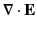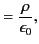(1)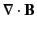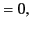(2)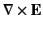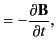(3)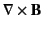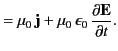(4)

Here,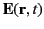,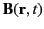,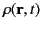, and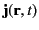represent the electric field-strength, the magnetic field-strength, the electric charge density, and the electric current density, respectively. Moreover,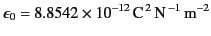(5)

is the electric permittivity of free space, whereas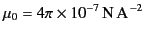(6)

is the magnetic permeability of free space. As is well known, Equation (1) is equivalent to Coulomb's law (for the electric fields generated by point charges), Equation (2) is equivalent to the statement that magnetic monopoles do not exist (which implies that magnetic field-lines can never begin or end), Equation (3) is equivalent to Faraday's law of electromagnetic induction, and Equation (4) is equivalent to the Biot-Savart law (for the magnetic fields generated by line currents) augmented by the induction of magnetic fields by changing electric fields.

Maxwell's equations are linear in nature. In other words, if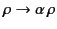and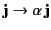, where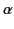is an arbitrary (spatial and temporal) constant, then it is clear from Equations (1)-(4) that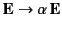and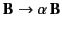. The linearity of Maxwell's equations accounts for the well-known fact that the electric fields generated by point charges, as well as the magnetic fields generated by line currents, are superposable.

Taking the divergence of Equation (4), and combining the resulting expression with Equation (1), we obtain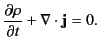(7)

In integral form, making use of the divergence theorem, this equation becomes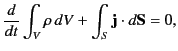(8)

where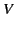is a fixed volume bounded by a surface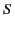. The volume integral represents the net electric charge contained within the volume, whereas the surface integral represents the outward flux of charge across the bounding surface. The previous equation, which states that the net rate of change of the charge contained within the volumeis equal to minus the net flux of charge across the bounding surface, is clearly a statement of the conservation of electric charge. Thus, Equation (7) is the differential form of this conservation equation.

As is well known, a point electric charge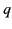moving with velocity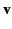in the presence of an electric field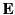and a magnetic field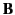experiences a force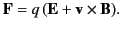(9)

Likewise, a distributed charge distribution of charge density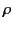and current density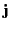experiences a force density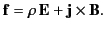(10)Next: Scalar and Vector Potentials Up: Maxwell's Equations Previous: Introduction
Richard Fitzpatrick 2014-06-27